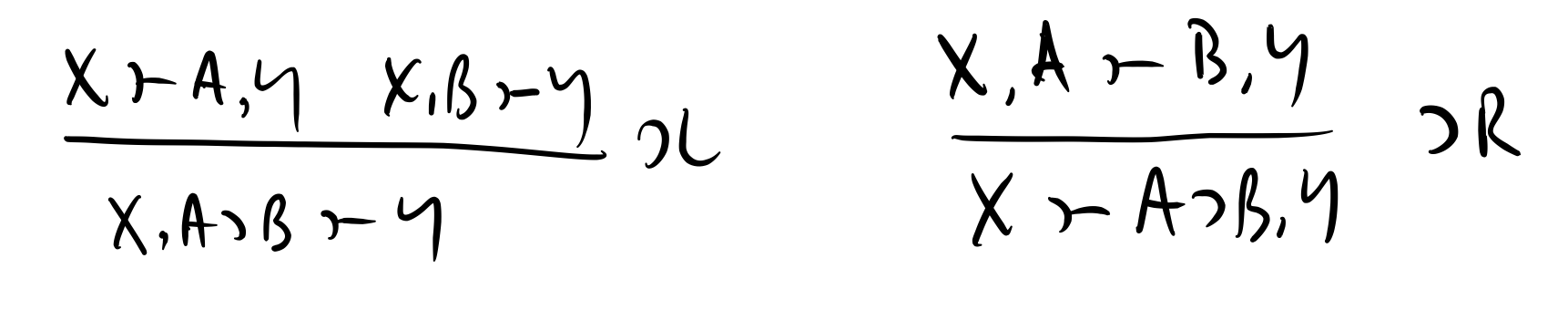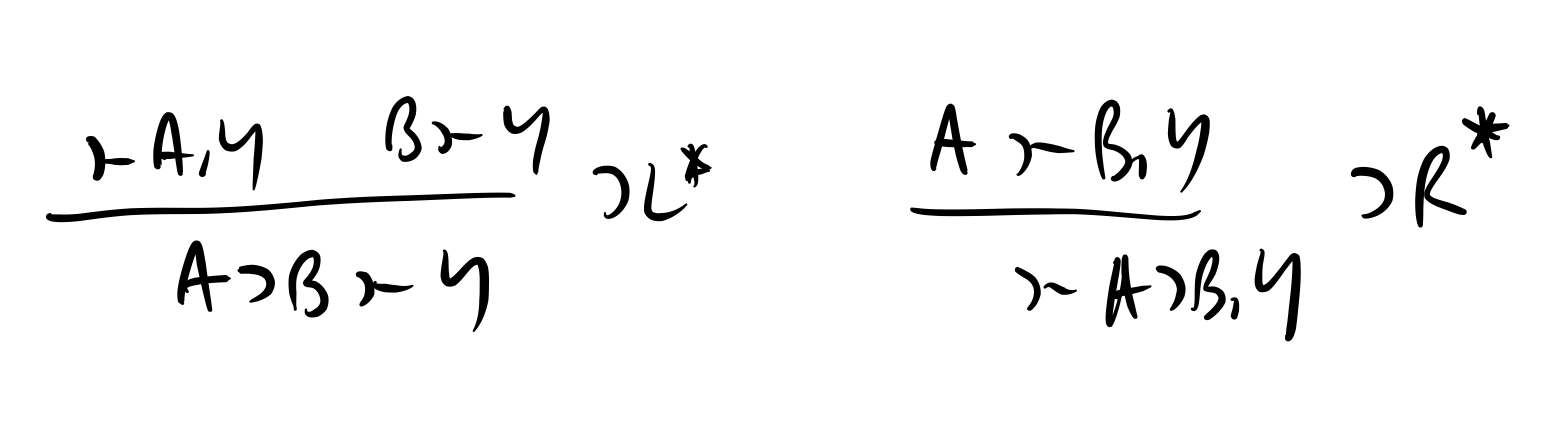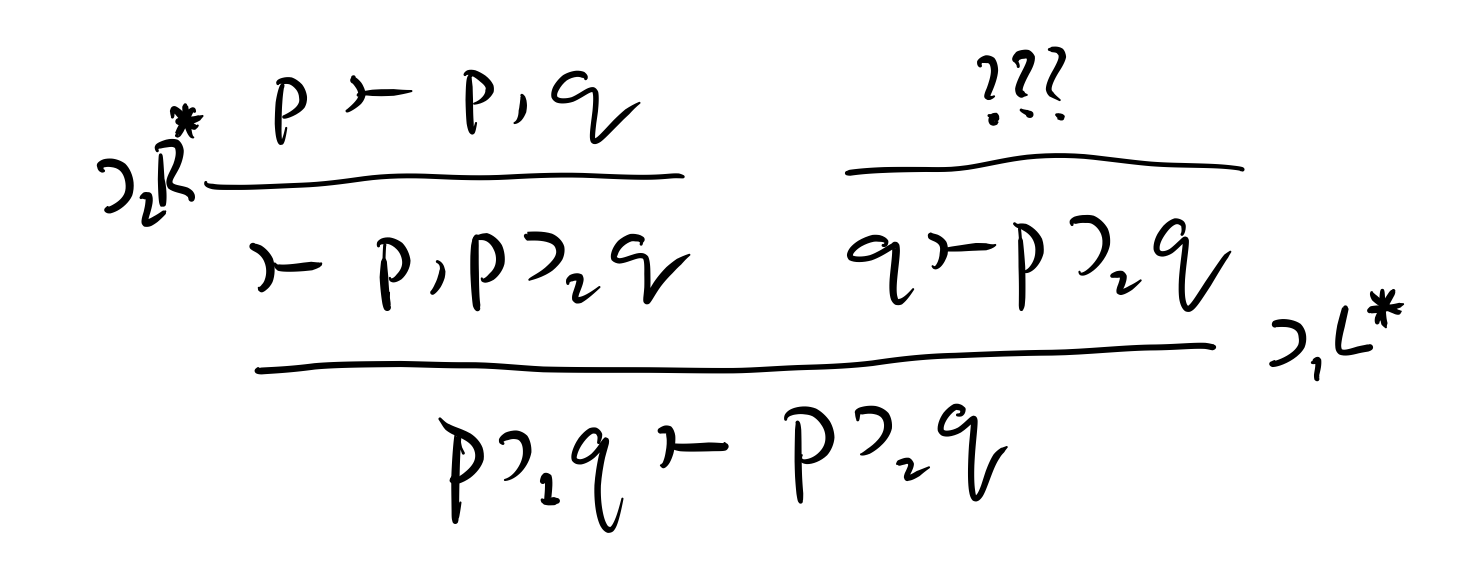## Conditionals in Closed Set Logic

#### July 22, 2017

Over the last couple of days on Twitter, I was involved in a thread, kicked off by Dan Piponi, discussing closed set logic—the natural dual of intuitionistic logic in which the law of the excluded middle holds but the law of non-contradiction fails, and which has models in the closed sets of any topological space, as opposed to the open sets, which model intuitionistic logic.

$$\def\ydash{\succ}$$This logic also has a nice sequent calculus in which sequents have one premise (or zero) and multiple conclusions. In the thread I made the claim that this is a natural and beautiful sequent calculus (it is!) but that the structure of the sequents means that the logic doesn’t have a natural conditional. The dual to the conditional (subtraction) can be defined, for which $$A\ydash B\lor C$$ if and only if $$A-B\ydash C$$. But the traditional conditional rules don’t work so well.

I realised, when I thought about it a bit more, that this fact is something I’ve just believed for the last 20 years or so, but I’ve never seen written down, so now is as good as a time, and here is as good as a place as any to explain what I mean.

Consider the conditional rules in the classical sequent calculus. They look something like this (give or take variations in the presentation, all equivalent given the classical structural rules):Classical sequent rules for the conditional.

If we restrict these rules to multiple conclusion single premise sequents, we get rules which look like these:Closed set logic sequent rules for the conditional.

You can see an immediate issue with the [$$\supset$$R*] rule. The concluding sequent is $$\ydash A\supset B,Y$$ which tells you when a conditional (with alternate conclusion cases) is derivable from no premises. It does not tell you anything else about when a conditional (with alternate conclusion cases) is derivable from another premise. It does not tell us what to do if we want to derive $$C\ydash A\supset B,Y$$ in any case where the $$C$$ is doing some logical work. The best guidance we get is to ignore the $$C$$ and to hope that we can derive $$\ydash A\supset B,Y$$. (In classical logic, that’s fine, because we could stash the $$C$$ premise away as an alternate conclusion $$\neg C$$ among the $$Y$$s in the right hand side, but in an asymmetric sequent calculus like this, that’s not necessarily within our powers.)

The fact that the rules seem too weak to constrain arbitrary sequents of the form $$C\ydash A\supset B,Y$$ gives us a hint that these rules might not be strong enough to actually characterise or uniquely define the connective $$\supset$$. And that hint bears out when you attempt to derive uniqueness. Here’s the issue. Imagine that you and I both use rules like these to define a conditional connective. Yours is $$\supset_1$$ and mine is $$\supset_2$$. Try to derive the sequent $$p\supset_1 q\ydash p\supset_2 q$$ and you’ll see that you get stuck:Attempted derivation of an identity sequent.

You get stuck at just the point where we’d like to know when $$p\supset_2 q$$ follows from other premises, and our rules give us no guidance at all. So, it looks as if our rules are not uniquely characterising in this sequent calculus.

That’s just a suspicion. It’d be nice to have a demonstration of this fact—an explanation of how it is that these rules could be interpreted in different, incompatible ways.

Here’s one way to show that the single premise / multiple conclusion conditional rules do not define a unique connective in closed set logic. We’ll use very simple algebras that are known to model closed set logic. Finite total orders. To be concrete, we’ll interpret propositions as taking values from some given subset of the interval $$[0,1]$$, at least including $$0$$ and $$1$$, so each formula $$A$$ will take some value $$a$$ in that set of values, and we’ll interpret a sequent $$A\ydash B_1,\ldots,B_n$$ as saying that $$a\le \max(b_1,\ldots,b_n)$$, which amounts to saying that $$a\le b_i$$ for some $$i$$. And similarly, $$\ydash B_1,\ldots,B_n$$ amounts to $$1\le b_i$$ for some $$i$$. A sequent holds if the value of the left hand formula (or $$1$$, if the formula is absent) is less than or equal to the value of one of the right hand formulas. (If the language has conjunction and disjunction, you can interpret them as $$\min$$ and $$\max$$ respectively, and the top and bottom values are $$0$$ and $$1$$.)

(What has this to do with closed set logic? An $$n+1$$ valued algebra corresponds to the closed sets in the topological space of an $$n$$-element totally ordered set where the closure of a set is its upwards closure in the ordering. $$0$$ is the empty set and $$1$$ is the whole space.)

Now, look at what the sequent rules for a conditional mean in this setting. Collapsing the finite set $$Y$$ in the rules to a single formula $$C$$ for simplicity’s sake (without any loss of generality), [$$\supset$$R*] tells us that if $$a\le\max(b,c)$$ then $$1\le\max(a\supset b,c)$$. That is, if $$a\le b$$ or $$a\le c$$ then either $$a\supset b=1$$ or $$c=1$$. This is to hold for all values for $$a$$, $$b$$ and $$c$$. A little bit of algebraic manipulation shows that this is equivalent to saying that when $$a\le b$$ or $$a\lt 1$$ then $$a\supset b=1$$.

And [$$\supset$$L*] tells us that if $$1\le \max(a,c)$$ and $$b\le c$$ then $$a\supset b\le c$$ for all $$a$$, $$b$$ and $$c$$. That is, if either $$1\le a$$ or $$1\le c$$ and $$b\le c$$ then $$a\supset b\le c$$. Some more manipulation tells shows that this holds if and only if $$1\supset b\le b$$.

So, [$$\supset$$L*] and [$$\supset$$R*] are satisfied in our order models when we have

• $$1\supset b\le b$$
• If $$a\le b$$ or $$a\lt 1$$ then $$a\supset b=1$$

And this is enough to fix many of the values of $$a\supset b$$, but it is nowhere near enough to fix all of them. They do tell us that $$a\supset b=1$$ whenever $$a\lt 1$$, and also, when $$a=b=1$$. But the values for $$1\supset b$$ are less constrained. For example these rules are satisfied by setting $$1\supset b =b$$ for all $$b$$. And they’re also satisfied by setting $$1\supset 1 = 1$$ and $$1\supset b=0$$ when $$b\lt 1$$. Provided that there’s at least one extra value between $$0$$ and $$1$$ in the ordering, that gives us wiggle room.

No wonder we couldn’t show that $$p\supset_1 q$$ entails $$p\supset_2 q$$! In this case (when $$p$$ takes the value $$1$$ and $$q$$ takes the value $$\frac{1}{2}$$) that inference takes us from $$\frac{1}{2}$$ to $$0$$. That sequent isn’t valid.

That’s what I meant when I said that the conditional rules were not so good in closed set logic. The rules tell us something about conditionals, but they are not specific or strong enough to characterise a single concept.

← Typesetting Flow Graphs with tikz | News Archive | Twelve things I love about philosophical logic →